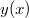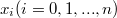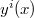ď»ż

### Introduzione

#### Abstract

En
By means of a modification to the Cook's method and by some quadrature formulas we obtain a class of methods that allow to smooth a function${y(x)}$,which is differentiable and known at the points$x_i (i=0,1,...,n)$ and to evaluate the numerical values taken at the same points by the derivative$y^i(x)$.

DOI Code: §

Full Text: PDFThis work is licensed under a Creative Commons Attribuzione - Non commerciale - Non opere derivate 3.0 Italia License.